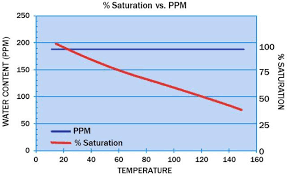## How to Calculate and Solve for Oil Saturation of Reservoir Fluid Flow | The Calculator EncyclopediaThe image above represents the oil saturation.

To compute for the oil saturation, five essential parameters are needed and these parameters are cumulative oil production (Np), oil in place (N), initial water saturation (Swi), initial oil FVF (Boi) and oil FVF (Bo).

The formula for calculating oil saturation:

S = [1 – Swi] [1 – Np / N] [Bo / Boi]

Where;

S = Oil Saturation
Np = Cumulative Oil Production
N = Oil in Place
Swi = Initial Water Saturation
Boi = Initial Oil FVF
Bo = Oil FVF

Let’s solve an example;
Given that the cumulative oil production is 22, oil in place is 8, initial water saturation is 16, initial oil FVF is 12 and the oil FVF is 7.
Find the oil saturation?

This implies that;

Np = Cumulative Oil Production = 22
N = Oil in Place = 8
Swi = Initial Water Saturation = 16
Boi = Initial Oil FVF = 12
Bo = Oil FVF = 7

S = [1 – Swi] [1 – Np / N] [Bo / Boi]
S = [1 – 16] [1 – 22/8] [7/12]
S = [1 – 16] [1 – 22/8] [0.583]
S = [1 – 16] [1 – 2.75] [0.583]
S = [1 – 16] [-1.75] [0.583]
S = [-15] [-1.75] [0.583]
S = 15.31

Therefore, the oil saturation is 15.31.